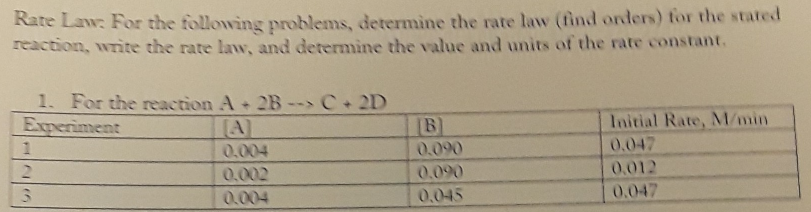# Rate Law: For the following problem, determine the rate law (find order) for the stated reaction, write the rate law, and determine the value and units of the rate constant. For the reaction A + 2B → C + 2D Experiment 1: [A] 0.004 [B] 0.090 [Initial Rate, M/min] 0.047 Experiment 2: [A] 0.002 [B] 0.090 [Initial Rate, M/min] 0.012 Experiment 3: [A] 0.004 [B] 0.045 [Initial Rate, M/min] 0.047